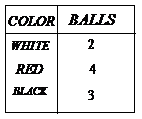A box contains two white, three black and four red balls. The number of ways in which we can select three balls from the box, if at least one black ball is to be included in the selection, is

# A box contains two white, three black and four red balls. The number of ways in which we can select three balls from the box, if at least one black ball is to be included in the selection, is

1. A
${2}^{6}$
2. B
${2}^{5}$
3. C
${2}^{7}-{2}^{6}$
4. D
none of these

Fill Out the Form for Expert Academic Guidance!l

+91

Live ClassesBooksTest SeriesSelf Learning

Verify OTP Code (required)

### Solution:$\therefore$  Number of ways $={\text{\hspace{0.17em}}}^{3}{C}_{1}×\text{\hspace{0.17em}}{\text{\hspace{0.17em}}}^{6}{C}_{2}+{\text{\hspace{0.17em}}}^{3}{C}_{2}×{\text{\hspace{0.17em}}}^{6}{C}_{1}+\text{\hspace{0.17em}}{\text{\hspace{0.17em}}}^{3}{C}_{3}×{\text{\hspace{0.17em}}}^{6}{C}_{0}$

$=64={2}^{6}$+91

Live ClassesBooksTest SeriesSelf Learning

Verify OTP Code (required)